# 【Python基础】第十四课：资料转换

## 向量化计算，Apply，Map，ApplyMap

Posted by x-jeff on July 8, 2020

# 2.向量化计算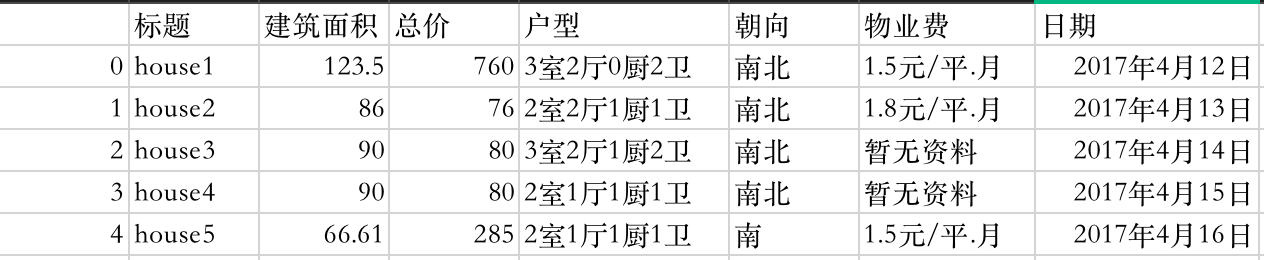1
2
import pandas as pd


1
2
3
4
#显示所有列
pd.set_option('display.max_columns', None)
#显示所有行
pd.set_option('display.max_rows', None)


👉“总价”一栏的单位是万元，将其转换成元：

1
df["总价"]*10000


👉把“朝向”和“户型”两栏进行合并：

1
df["朝向"]+df["户型"]


👉建立新的栏位“均价”：

1
df["均价"]=df["总价"] * 10000 / df["建筑面积"]


# 3.Apply、Map、ApplyMap

• Map：将函数套用到Series上的每个元素。
• Apply：将函数套用到DataFrame上的行与列。
• ApplyMap：将函数套用到DataFrame上的每个元素。

## 3.1.Map

👉移除“物业费”中的元：

1
2
3
def removeDollar(e):
return e.split('元')
df["物业费"].map(removeDollar)


split用法👉：split

1
2
3
s="1.5元/平米.月"
s.split("元")#返回一个列表['1.5', '/平米.月']
s.split("元")#返回1.5


1
df["物业费"].map(lambda e:e.split('元'))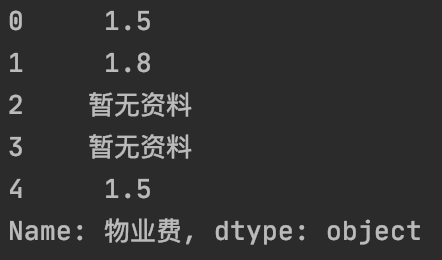## 3.2.Apply

1
df2=pd.DataFrame([[60,70,50],[80,79,68],[63,66,82]],columns=["First","Second","Third"])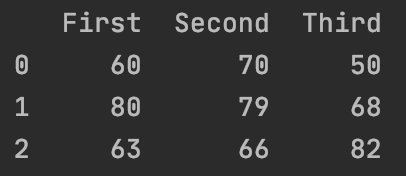1
2
df2.apply(lambda e:e.max()-e.min(),axis=0)#默认为axis=0
df2.apply(lambda e:e.max()-e.min(),axis=1)


axis=0时按列输出为：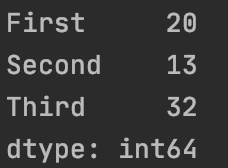axis=1时按行输出为：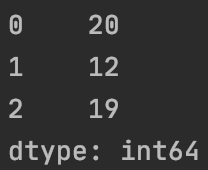## 3.3.ApplyMap

1
2
3
4
5
6
7
8
9
#方法一
def convertNaN(e):
if e == "暂无资料":
return np.nan
else:
return e
df.applymap(convertNaN)
#方法二
df.applymap(lambda e:np.nan if e=="暂无资料" else e)


# 4.代码地址